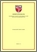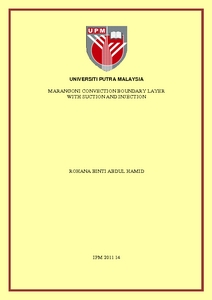Simple Search:

# Marangoni convection boundary layer with suction and injection

## Citation

Abdul Hamid, Rohana (2011) Marangoni convection boundary layer with suction and injection. Masters thesis, Universiti Putra Malaysia.

## Abstract / Synopsis

Marangoni convection boundary layer has become of noticeable importance in recent years. Its influential role in numerous engineering applications such as crystal growth and circuit manufacture tends to attract the researchers. In this study, we have numerically discovered the effects of suction and injection on the Marangoni forced convection boundary layer in the presence of many other parameters such as thermal radiation, Joule heating, viscous dissipation and magnetic field. The continuity equation, the momentum equation and the energy equation with the governing parameter of interest are transformed into the ordinary differential equations using similarity transformation. The transformed equations are then solved using the shooting method and Runge-Kutta-Fehlberg method in the Maple programming. Numerical results obtained in this study are the interface velocity, the heat transfer rate at the wall, the velocity profiles as well as the temperature profiles. From the results, it is noticed that the parameters can be used to control the Marangoni convection boundary layer flow. The Joule heating, viscous dissipation, magnetic field, thermal radiation and suction parameters have the effects to increase the thermal boundary layer thickness, thus reduce the heat transfer at the interface.Preview
PDF
IPM 2011 14R.pdfView Item